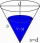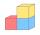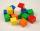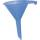# Triangle + cube root - math problems

#### Number of problems found: 11

• The funnelThe funnel has the shape of an equilateral cone. Calculate the content of the area wetted with water if you pour 3 liters of water into the funnel.
• Cube diagonalsCalculate the length of the side and the diagonals of the cube with a volume of 27 cm3.
• Body diagonalCalculate the cube volume, whose body diagonal size is 75 dm. Draw a picture and highlight the body diagonal.
• Pyramid cutWe cut the regular square pyramid with a parallel plane to the two parts (see figure). The volume of the smaller pyramid is 20% of the volume of the original one. The bottom of the base of the smaller pyramid has a content of 10 cm2. Find the area of the
• CalculateCalculate the length of the wall diagonal of the cube with an edge 5 cm long.
• Cube and sphereCube with the surface area 150 cm2 is described sphere. What is sphere surface?
• Cube - wallV kocke ABCDEFGH je ?. Aký je povrch kocky?
• FunnelThe funnel has the shape of an equilateral cone. Calculate the surface wetted with water if we poured into the funnel 7.1 liters of water.
• Rotary coneThe volume of the rotation of the cone is 472 cm3, and the angle between the side of the cone and the base angle is 70°. Calculate the lateral surface area of this cone.
• CubesOne cube is an inscribed sphere and the other one described. Calculate the difference of volumes of cubes, if the difference of surfaces in 257 mm2.
• Cube diagonalDetermine the length of the cube diagonal with edge 37 mm.

We apologize, but in this category are not a lot of examples.
Do you have an exciting math question or word problem that you can't solve? Ask a question or post a math problem, and we can try to solve it.

We will send a solution to your e-mail address. Solved examples are also published here. Please enter the e-mail correctly and check whether you don't have a full mailbox.

See also our trigonometric triangle calculator. Triangle Problems. Cube root - math problems.